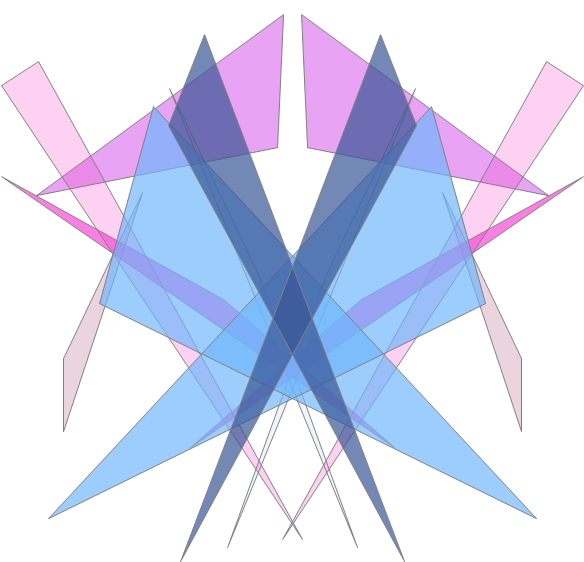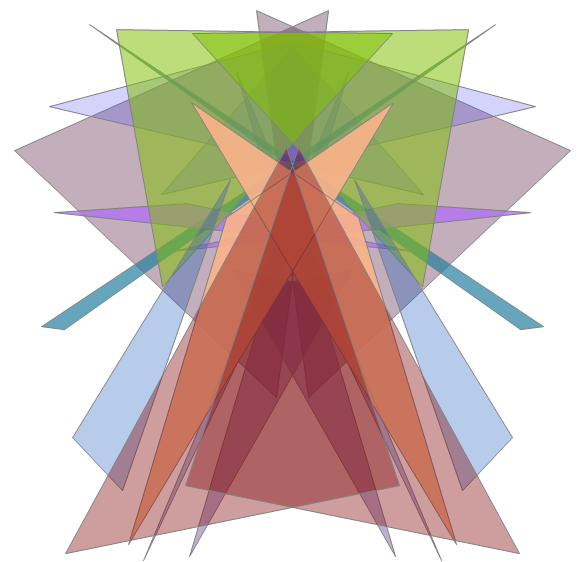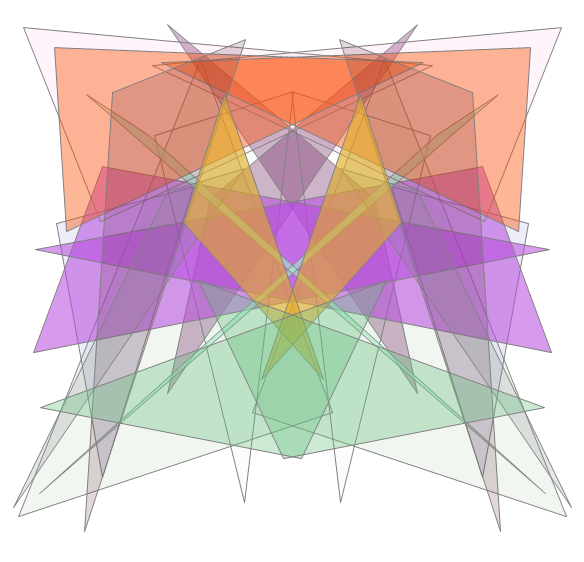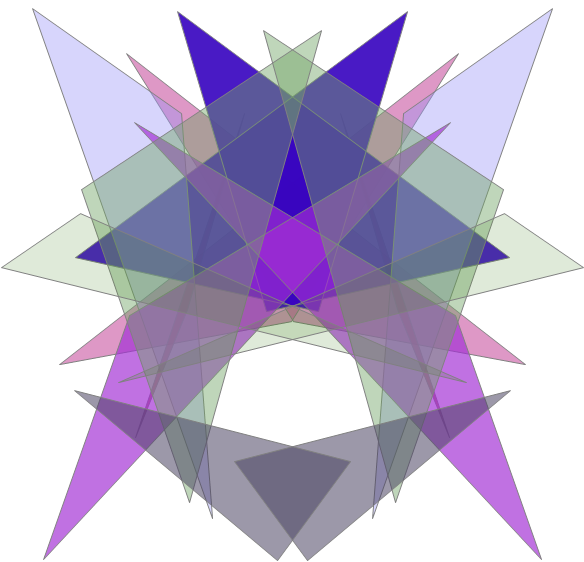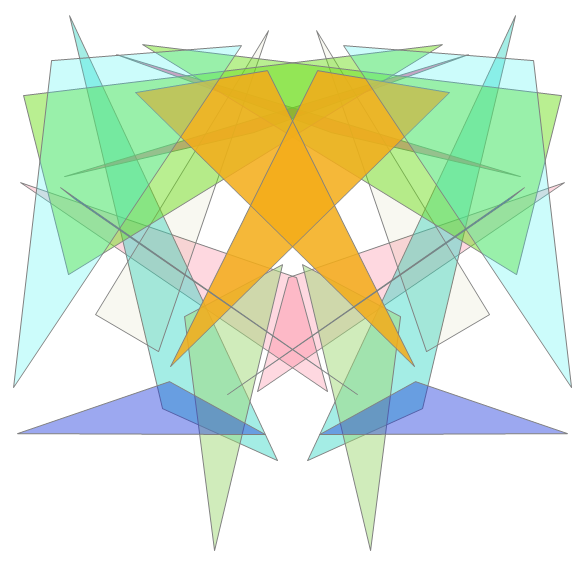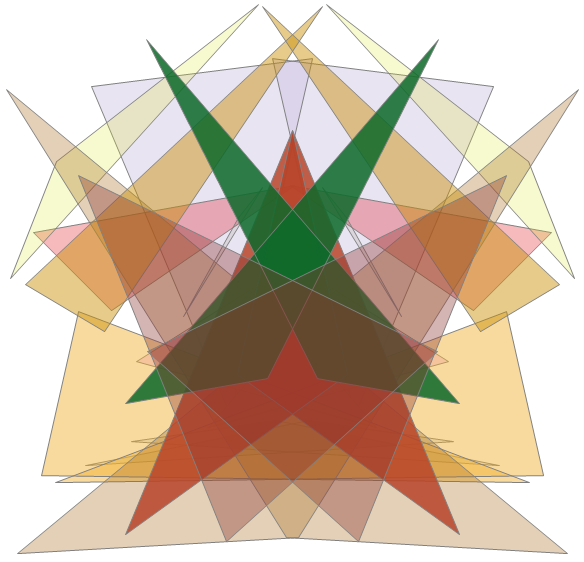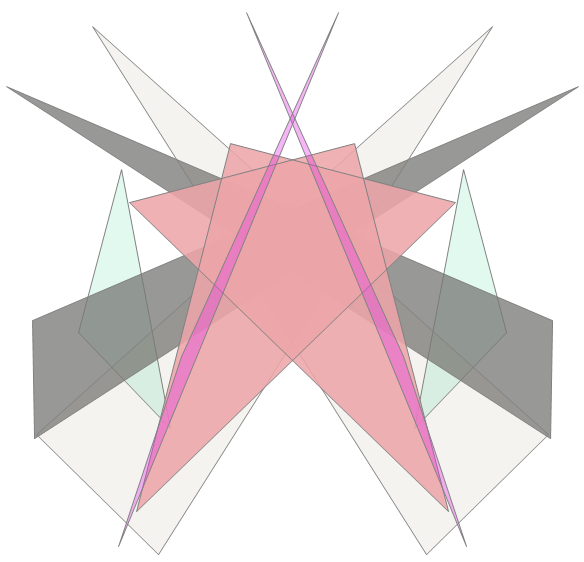Variable Package: cg ToC DocOverview CGDoc RelNotes FAQ Index PermutedIndex
Allegro CL version 10.0

## *alpha-blending*

This variable has an effect only on the Windows platform.

This variable can be used to create translucent effects when drawing lines, filling areas, and displaying pixmaps, where anything that was drawn earlier appears to partially "show through" new content that is drawn later.

When the value is true, a drawing or filling function such as draw-line or fill-polygon will use the alpha component of the current foreground-color that is being used for the drawing. See make-rgb, where the alpha component of a color can be specified. When displaying pixmaps, if the alpha argument is passed to copy-to-stream then it will be used whenever the value of this variable is true.

An alpha value is an integer between 0 and 255 inclusive. A value of 255 represents full opacity, where the pre-existing background does not show through at all, and is no different than drawing without alpha blending. A value of 0 represents full transparency, where the new content is not seen at all (and so of course is not useful). A typical value might be 192, which will blend three-fourths of the color that's being drawn with one-fourth of the colors that the pixels had before. This lets the underlying image show through a little while mostly showing the new content in front of it.

The default value is `nil` for backward compatibility and efficiency. Typically you would bind this variable to true around a block of drawing code where alpha blending is desired. Or this variable could simply be set to true globally if the effect is desired everywhere.

Caveat: When using alpha-blending, any existing background at all will be blended with the content that's being drawn. Even with a fairly large alpha value like 192 (for three-fourths opacity), background areas that are white will still show through by one-fourth, making the content that's being drawn appear somewhat washed-out. And new content that's drawn over black areas of the background will be darker than they would be without alpha-blending. This may limit the usefulness of alpha blending to special effects, rather than where you want the content that's being drawn in front to look as good as it can look by itself.

See `*antialiasing*` and `*color-gradient-filling*` for other appearance-enhancing effects.

## Example

This example simply generates a set of random triangles and fills them with random colors that use random amounts of alpha blending, and adds bilateral symmetry. Clicking the window generates another image. The alpha blending works only on the Windows platform, but the code runs elsewhere.

```
(defclass triangle-window (frame-window)
((triangles :accessor triangles :initform nil))
(:default-initargs
:double-buffered t
:scrollbars nil
:width 600 :height 600))

(defmethod make-triangles ((window triangle-window))
(let* ((width (interior-width window))
(height (interior-height window))
triangles vertices mirrors x y color)
(dotimes (j (+ 3 (random 12)))
(setq vertices (make-array 3))
(setq mirrors (make-array 3))
(dotimes (k 3)
(setq x (random width))
(setq y (random height))
(setf (aref vertices k)(make-position x y))
(setf (aref mirrors k)(make-position (- width x) y)))
(setq color (make-rgb :red (random 255) :green (random 255)
:blue (random 255) :alpha (random 255)))
(push (list (list color vertices)
(list color mirrors))
triangles))
(setf (triangles window) triangles)))

(defmethod redisplay-window ((window triangle-window) &optional box)
(declare (ignore box))
(clear-page window)
(unless (triangles window)
(make-triangles window))
(let* ((*antialiasing* t)
(*alpha-blending* t))
(dolist (pair (triangles window))
(dolist (triangle pair)
(with-foreground-color (window (first triangle))
(fill-polygon window (second triangle))))
(dolist (triangle pair)
(with-foreground-color (window gray)
(draw-polygon window (second triangle)))))))

(defmethod mouse-left-down ((window triangle-window) buttons position)
(declare (ignore buttons position))
(make-triangles window)
(invalidate window))

(make-window :triangle-window
:class 'triangle-window)
```

Here are some pictures showing triangles with alpha blending.